• 0

# Graphical Summary for Discrete Data

Bar Chart is a graphical tool used to summarize discrete data. Each bar can represent count, function (such as the mean, sum, or standard deviation), or summary of values of a category.

Pie Chart is a graphical tool used to summarize discrete data by displaying the proportion of each data category relative to the whole data set.

An application-oriented question on the topic along with responses can be seen below. The best answer was provided by Himanshu Sharma on 29th Nov 2022.

Applause for all the respondents - Gulshan Kumar, Suresh Kumar Gupta, Himanshu Sharma.

## Question

Q 525. What are the charts used for graphical summary of Discrete data? Are there any specific use cases where only a particular chart is used while others cannot be used? Participant providing maximum number of relevant use cases will be considered as the winner.

Note for website visitors - Two questions are asked every week on this platform. One on Tuesday and the other on Friday.

## Recommended Posts

• 1

Background: Quantitative data is divided into two categories – Discrete and continuous data. As No. of water drops is Discrete but volume of water is continuous.

So when data is countable and can take certain value it is called Discrete data, whole no is used to write Discrete data, Example – Number of student in a class, Number of player in cricket team, Number of Defect per day, Number of books in Book shelf etc. Following type of Graph can be used to represent summery of discrete data:

1-   Frequency table

2-   Bar Chart

3-   Pie Chart

4-   Pareto Chart

In Frequency table values are represented thorough tally mark representing frequency of data. Below example shows defect monitoring in injection moulding process. Tally mark helps in live data monitoring to avoid end of shift reconciliation. Summery of data can be used for different type of bar chart.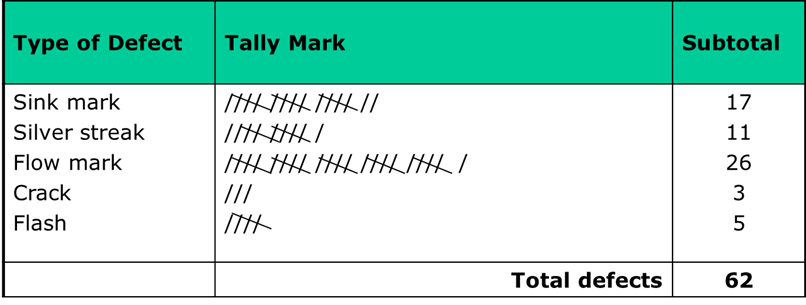Bar chart use to do following:

1-   Compare counts by category:

2-   Display a frequencies on y axis

3-   Understand relationship between categorical variable.

In Below Example: Bar chart shows Summary of defects on X axis and defect frequencies on Y axis - help to visualize defect wise frequency as shown in below example: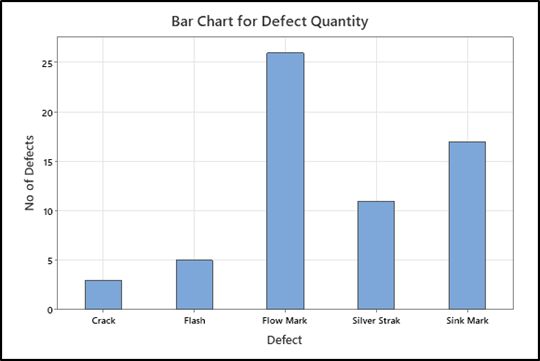In Minitab and Excel many option are available for bar charts based on requirement. Below options are available in Minitab: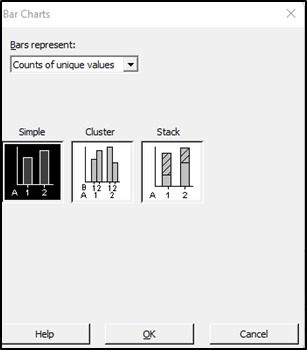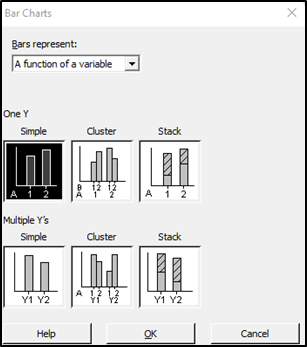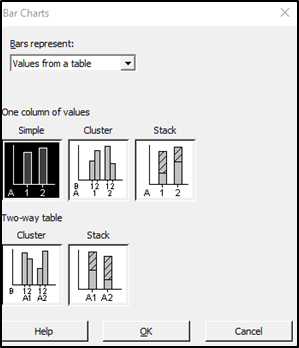Cluster Bar chart showing comparison between Male and Female and preferences for ice cream flavour as shown below: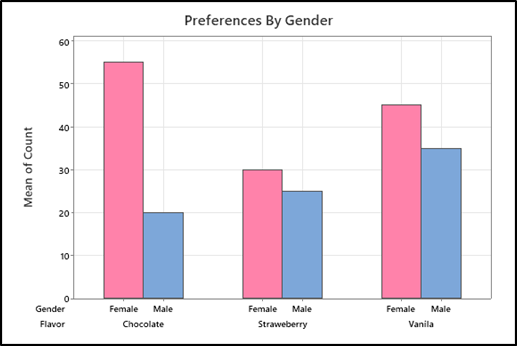Another Example:  Pizza delivery service promise to deliver pizza in specified time. Delivery status compared with time as peak and non-peak.

Below possible outcome can be seen using bar graph that peak time having more late deliveries compares to non-peak time.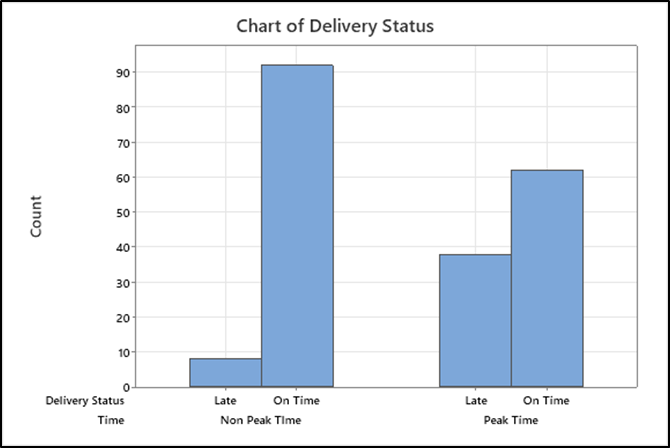Bar chart for Ordinal data:  Service quality compared on 5 point Likert scale for customer feedback. As below:

1-   Very Good

2-   Good

3-   Average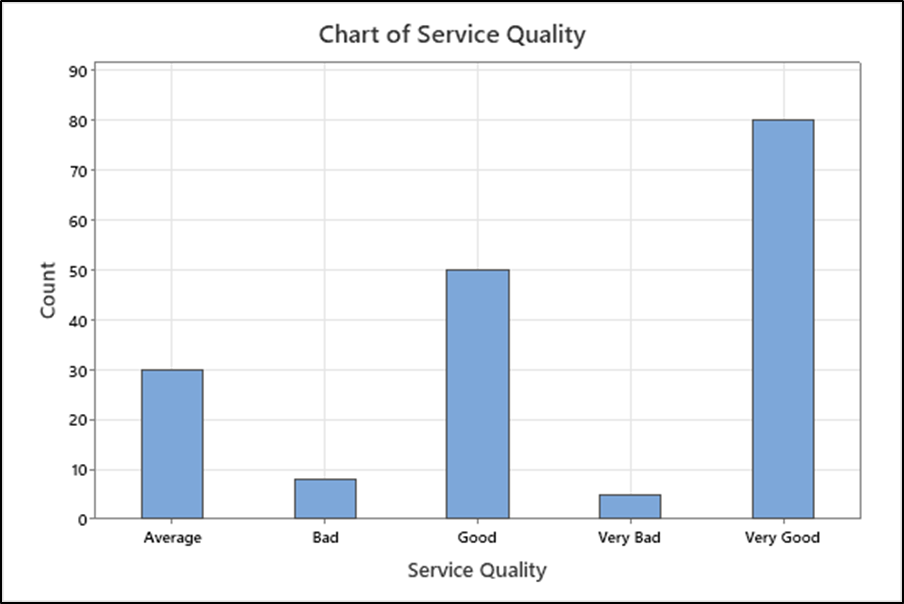Pie Chart: We can also make Pie Chart as shown below for defects to see frequency or proportion distribution for of each defect: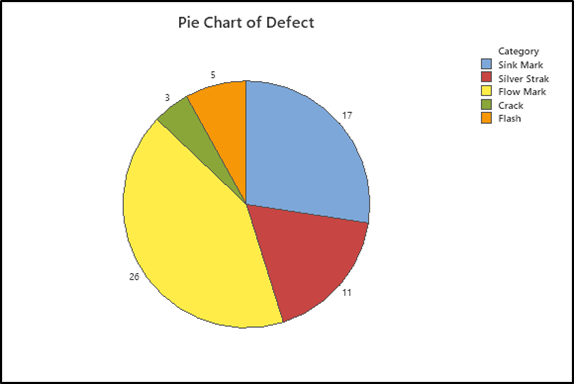Pareto Chart is combination of line and bar graph and used to prioritize focus area for project.Below pareto chart shows Flow mark contribute maximum as 41.9% and flow chart and sink mark contribute 69.7%. It shows frequency of defects on primary axis and cumulative percentage on secondary axis. in Below Example X Axis and Primary Y axis using discret data and secondery Y axis having variable data.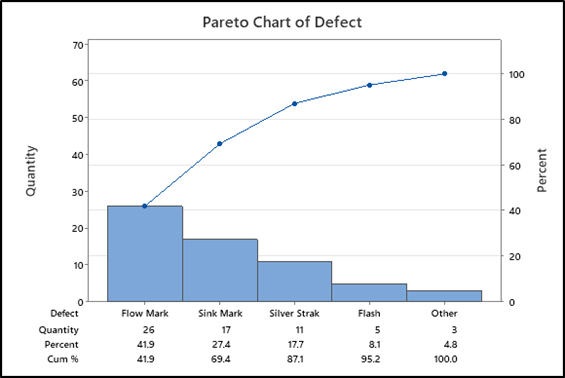Above example can be used to present graphical summery of discrete data and required inferance can be drawn.

##### Share on other sites

• 0Discrete data is a count that involves integers. Only a limited number of values is possible in case of Discrete data . It cannot be subdivided into parts.E.g., the number of children or teachers in a school or workers in a factory is discrete data. They cannot be further broken down or subdivided into fractions .

Examples of discrete data:

Ø  Number of students in  class.

Ø  Number of workers in  company.

Ø  The number of parts damaged during transportation.

Ø  Shoe sizes.

Ø  Number of languages an individual speaks.

Ø  The number of test questions you answered correctly.

Ø  Instruments in a shelf.You can count the data.

The values cannot be divided into smaller pieces rendering the data futile.

You cannot measure the data. By nature, discrete data cannot be measured at all.Eg weight cannot be counted but measured .Hence Weight is Continuous Data.Other example is Revenue.

Possible values are limited e.g. days of the month.Discrete data may be ordinal or nominal data :

ü  When there is an order or rank to the values, we have ordinal discrete data. For example, the first, second and third person in a ticket counter line.Graded or Stratified Data.

ü  Discrete data may be nominal where is no order in data between the values.Eg, the eye color may be one of these categories: blue, green, brown.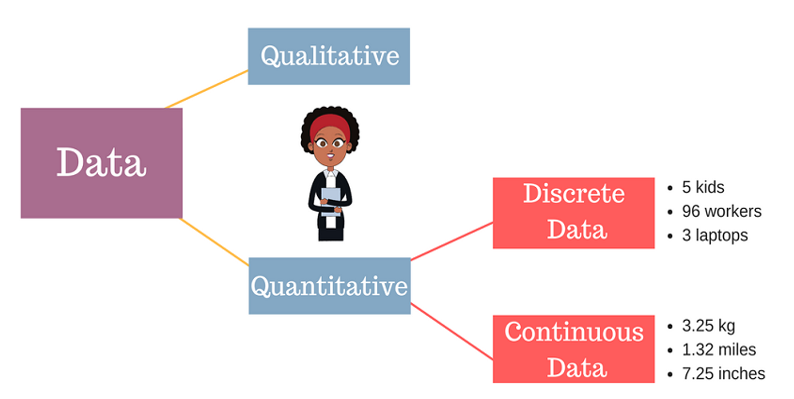The following charts are usually used for representing the discrete data:

v  BAR CHART.

v  STACKED BAR CHART.

v  COLUMN CHART.

v  STACKED COLUMN CHART.

v  SPIDER CHART.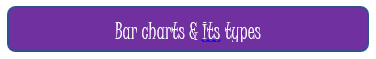A bar graph is a specific method of representing data using rectangular bars where the dimensions specifically length of each bar is proportional to the value of the parameter they represent. Graphical representation of data using bars of different dimensions specifically height.

Properties of Bar Graph

ü  All rectangular bars should have equal dimensions and equal spacing in between.

ü  The rectangular bars are horizontally or vertically plotted.

ü  The height of the rectangular bar is equivalently proportional to the data they represent.

ü  The rectangular bars must be on a common base or parameter they represent.

Uses of Bar Graph

ü  The comparisons between different variables are easy, convenient and graphically visual.

ü  It is the easiest chart to prepare.

ü  Most widely used method of data presentation.

ü  It is used to compare data sets which are independent of one another.

ü  It helps in analysing patterns for longer period of time.

Types of Bar Graphs

Bar Graphs are chiefly classified into the following two types:

v  Vertical Bar Graph

v  Horizontal Bar Graph

Apart from the vertical and horizontal bar graphs, two more types of bar graphs are plotted and in use, which are given below:

Vertical Bar Graphs

When the given data is represented vertically in a graph or chart with the help of rectangular bars, such graphs are known as vertical bar graphs. The rectangular bars are vertically drawn on the x-axis parallel to the Y-axis and perpendicular to the X-axis, and the y-axis shows the value of the height of the rectangular bars.

Horizontal Bar Graphs

When the given data is represented horizontally parallel to the X-axis by using rectangular bars that show the measure of data, such graphs are known as horizontal bar graphs. The length of the bars in this type of charts also is equal to the values of different parameters.

Stacked Bar Graph

The stacked bar graph is also known as the COMPOSITE BAR GRAPH. It splits the entire length of the bar into different parts or groups. In this, each part of a bar is represented using different colours to easily identify the different parameters under observation. It requires specific labelling to indicate the different parts of the bar. Can be plotted as  both vertical and horizontal diagrams.

Grouped Bar Graph

The grouped bar graph is also known as the CLUSTERED BAR GRAPH. In this, rectangular bars are grouped by position representing the value of individual parameters with the same colours showing same parameters level within each group and then a comparative analysis is done for each parameter visually and data based . Can be plotted as  both vertical and horizontal diagrams.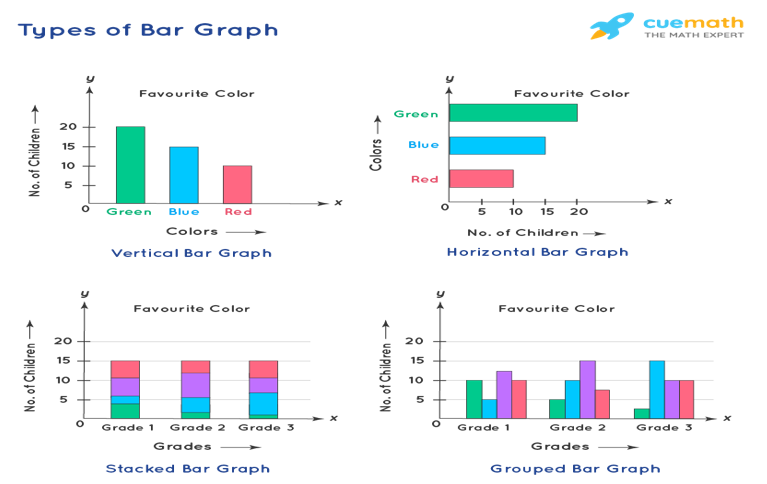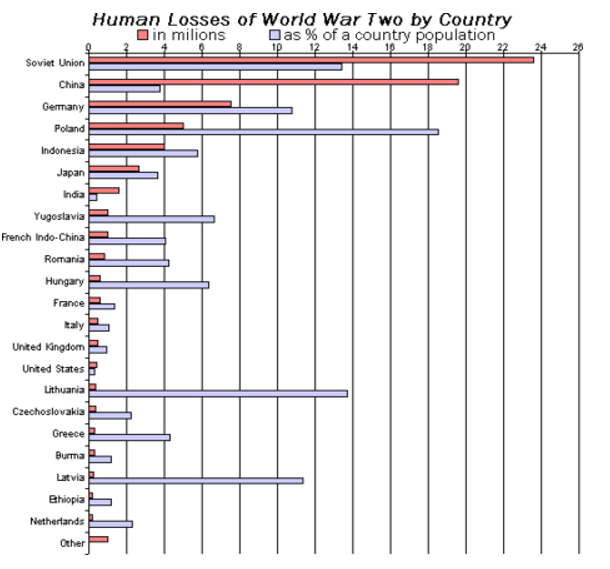Businesses use both bar graphs and pie charts to present information, such as sales ,revenue, profit etc, to customers as well as to employees and other businesses. People can also use bar graphs and pie charts for keeping track of finances etc. It is mostly used for comparison of two independent data sets.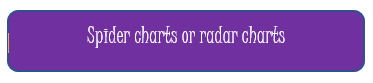A spider chart is a two-dimensional chart type designed to plot one or more series of values over multiple discrete variables. The spider chart is also known as radar chart ,web chart, spider graph, spider web chart, star chart, star plot, cobweb chart, irregular polygon, polar chart, or Kiviat diagram.

ü  Radar charts can be used in sports to chalk out players' strengths and weaknesses in various parameters.

ü  Another application of radar charts is the control of quality improvement to display the performance metrics of various objects including computer programs, computers, phones, vehicles, and more.

ü  Radar charts can be used in pharmaceutical industries to display the strengths and weakness of drugs and other medications.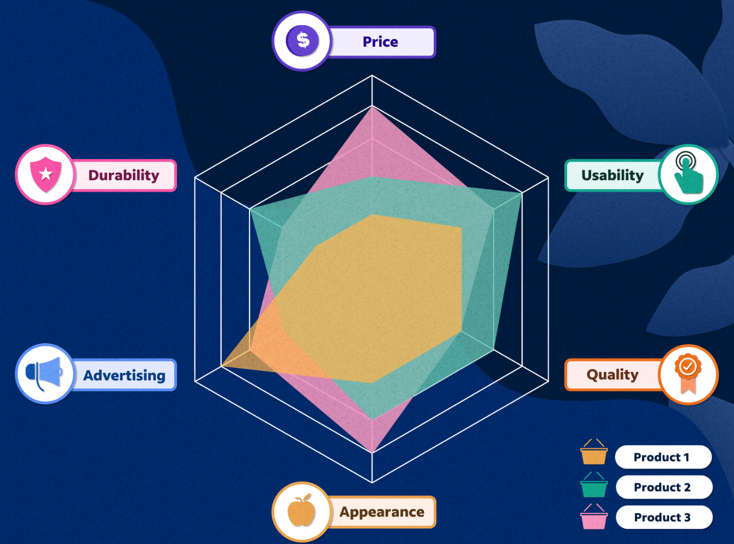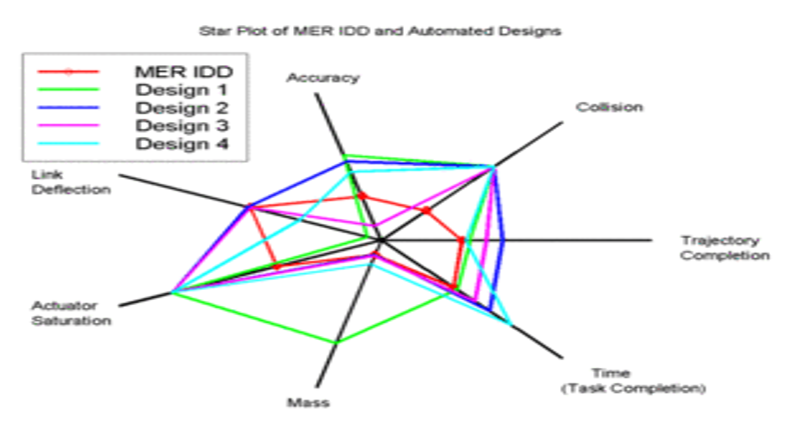##### Share on other sites

• 0

Discrete data is data that only takes certain values, data that can be counted and has a finite number of values.

Example: Errors count, Hourly Volume processed, No of Students in class etc. which can be counted

Below charts can be used for Graphical summary of Discrete Data: -

·  Column Chart - The most commonly used chart type for discrete data is the column chart. Every column in the chart represent one Value and column height denotes the frequency of the Data value. Example Comparison of monthly employee sales

We also have Stacked Column chart used for data composed of multiple column data series stacked on top of one another and is used to compare totals and notice changes at the item level

·  Bar Chart - Bar charts can also be used for discrete values. Bar charts are represented in horizontal bars and are often a better choice when we have long text labels which cannot be represented well in Column chart. Example A single-series chart in which the values are compared inside one category.

We also have Stacked Bar chart composed of multiple bar series stacked horizontally one after the other which makes it easier to track the variation in the individual values and their total value.

·  Pie Chart - Pie Chart also works well for displaying discrete data, as all the values combined equal 100 percent of the total. If you want to display your values as percentages of the total, then Pie Chart is best option. Pie charts is not recommended if there are too many small values as it becomes difficult for the reader to make sense of the chart.

· Spider Chart - It’s designed for comparing multivariate data that has three or more quantitative variables. Axes for each variable start from center point and are arranged radially around it like a spider web. It’s the process of plotting a polygon. Example - Employee Skill Assessment

· Histogram - When you have a large set of discrete data that doesn't fit on a regular chart, you can use a histogram to display the distribution of the values.

##### Share on other sites

• 0

Interesting answers to a seemingly simple question. The best answer has been provided by Himanshu Sharma.

##### Share on other sites

• 0

Discrete and Continuous Data

 s.no Discrete Data Continuous Data 1. Categoric ü Colors ü Gender ü Region ü Satisfaction Scale Ø Dimensions Ø Profit Ø Sales Revenue Ø Handle Time Ø Lead Time Ø Turn Around Time Ø Weight Ø Temperature 2. Count ü No. of Tickets ü No of Defects ü No of Clicks ü No of Items Sold

Discrete Data is best represented. using Bar Chart which displays grouped data using rectangular bars with lengths that are proportional to the values. Bar Charts are broadly used in marketing and finance.

there are other charts also used for Discrete Data.

1. Stacked Bar Chart- use it if you are interested in the proportional contributions either of categories to the total or of values within each category.

2. Column Chart- use it to track changes in values over time by comparing total column lengths.

3. Staked Column Chart is designed to compare total and notice changes at the item level that are likely to have the most impact

4. Bullet Chart- use for tracking progress towards goal and measuring how far you are from the target,

5. Pie Chart- it represents the data in circular graph.it requires a list of categorical variables and numerical variables. The term "Pie:" represents the whole, and the "slices" represent the parts of the whole.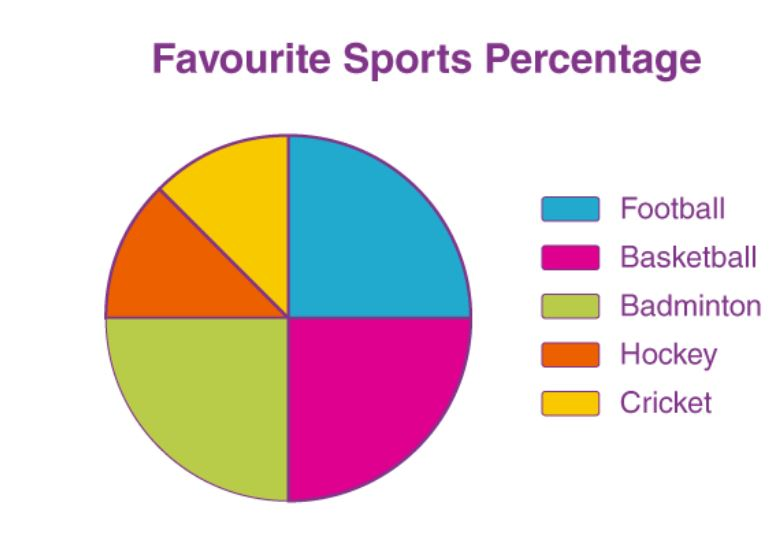## Create an account

Register a new account

• ### Who's Online (See full list)

• There are no registered users currently online
• ### Forum Statistics

• Total Topics
3.1k
• Total Posts
15.8k
• ### Member Statistics

• Total Members
54,460
• Most Online
990

×
×
• Create New...